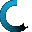Retrieving data ...
Please do not click anywhere else.

If you see no response after 2-3 minutes, then click on the [X] button in the upper right corner to close the window and retry the operation at a later time.
Interactive Multimedia Learning & Teaching Management System Suite## Course Contents

Course Name: 6th Grade Math (New)
• 1. Fractions, Decimal Numbers and Percentages
•  1.1 Converting Fractions, Decimal Numbers & Percentages
•  1.1.1 Fractions Review
•  1.1.2 Decimal Numbers Review
•  1.1.3 Percentages Review
•  1.1.4 Converting Fractions into Decimal Numbers
•  1.1.5 Converting Decimal Numbers into Fractions
•  1.1.6 Converting Decimal Numbers into Percents
•  1.1.7 Converting Percents into Decimal Numbers
•  1.1.8 Converting Percents into Fractions
•  1.1.9 Converting Fractions to Percents
•  1.2 Operations with Fractions and Decimal Numbers
•  1.2.1 The Greatest Common Factor
•  1.2.2 The Least Common Multiple
•  1.2.3 The Least Common Denominator
•  1.2.6 Subtracting Fractions
•  1.2.7 Subtracting Decimal Numbers
•  1.2.8 Multiplying Fractions
•  1.2.9 Multiplying Decimal Numbers
•  1.2.10 Dividing Fractions
•  1.2.11 Dividing Decimal Numbers
•  1.3 Operations with Mixed Numbers
•  1.3.1 Mixed Numbers Review
•  1.3.2 Converting Mixed Numbers into Fractions
•  1.3.3 Converting Fractions into Mixed Numbers
•  1.3.5 Subtracting Mixed Numbers
•  1.4 Percents, Fractions, and Decimals in Everyday Life
•  1.4.1 Working with Percents in Everyday Life
•  1.4.2 Working with Fractions in Everyday Life
•  1.4.3 Working with Decimal Numbers in Everyday Life
• 2. Positive and Negative Numbers
•  2.1 Positive and Negative Numbers Review
•  2.2 Adding Positive and Negative Numbers
•  2.3 Subtracting Positive and Negative Numbers
•  2.4 Multiplying Positive and Negative Numbers
•  2.5 Dividing Positive and Negative Numbers
• 3. The Number Line and Absolute Value
•  3.1 Number Line Review
•  3.2 Comparing and Ordering Numbers on a Number Line
•  3.3 Comparing and Ordering Fractions on a Number Line
•  3.4 Comparing and Ordering Decimals on a Number Line
•  3.5 Absolute Value Review
•  3.6 Finding the Absolute Value
•  3.7 Finding the Distance using the Absolute Value
• 4. Ratios and Proportions
•  4.1 Introduction to Ratios
•  4.2 Equivalent Ratios
•  4.3 Ratios in Simplest Form
•  4.4 Finding Ratios in Simplified Form
•  4.5 Introduction to Proportions
•  4.6 Finding the Missing Number in a Proportion
• 5. Exponents, Roots and Scientific Notation
•  5.1 Introduction to Exponents
•  5.2 Basic Rules of Exponents
•  5.3 Laws of Exponents
•  5.4 Negative Exponents
•  5.5 Squares and Square Roots
•  5.6 Cubes and Cube Roots
•  5.7 Simplifying Square Roots
•  5.8 Fractional Exponents
•  5.9 Simplifying Exponential Expressions
•  5.10 Evaluating Exponential Expressions
•  5.11 Powers of 10
•  5.12 Understanding Scientific Notation
• 6. Algebra - Variables
•  6.1 Variables Review
•  6.2 Like and Unlike Terms
•  6.3 Canceling Variables
•  6.5 Subtraction with Variables
•  6.6 Multiplication with Variables
•  6.7 Division with Variables
•  6.8 Expressions and Equations
•  6.9 Writing Expressions and Equations
•  6.10 Word Problems with Variables
•  6.11 Evaluating Expressions with Variables
• 7. Algebra - Real Numbers
•  7.1 Real Numbers Review
•  7.2 Properties of Real Numbers
•  7.3 Order of Operations
• 8. Algebra - The Coordinate Plane
•  8.1 The Coordinate Plane
•  8.2 Graphing Points on a Coordinate Plane
•  8.3 Graphing Linear Relations
•  8.4 Graphing Linear Relations using a t-Table
•  8.5 Making Predictions from Linear Patterns
• 9. Algebra - Linear Equations and Formulas
•  9.1 Introduction to Linear Equations
•  9.2 Writing Equations and Formulas
•  9.3 Problem Solving using Formulas
•  9.4 Linear Equations with One Variable
•  9.5 Writing Linear Equations with One Variable
•  9.6 Solving Linear Equations with One Variable
•  9.7 Linear Inequalities with One Variable
•  9.8 Solving Linear Inequalities with One Variable
• 10. Geometry - Angles and Polygons
•  10.1 Angles Review
•  10.2 Polygons Review
•  10.3 Complementary Angles
•  10.4 Supplementary Angles
•  10.5 Vertical Angles
•  10.6 Triangles
•  10.8 Finding Missing Angles - Part A
•  10.9 Finding Missing Angles - Part B
• 11. Geometry - Circles and Solid Shapes
•  11.1 The Circle
•  11.2 Circumference of a Circle
•  11.3 Area of a Circle
•  11.4 Spheres
•  11.5 Surface Area of a Sphere
•  11.6 Volume of a Sphere
•  11.7 Cylinders
•  11.8 Surface Area of a Cylinder
•  11.9 Volume of a Cylinder
•  11.10 Rectangular and Triangular Prisms
•  11.11 Surface Area of Rectangular and Triangular Prisms
•  11.12 Volume of Rectangular and Triangular Prisms
• 12. Measurements and Conversions
•  12.1 Systems of Measurement
•  12.2 Metric Numbers
•  12.3 Time
•  12.4 Mass and Weight
•  12.5 Length
•  12.6 Temperature
•  12.7 Volume
•  12.8 Rate of Speed
•  12.9 The Rate, Time and Distance Traveled
•  12.10 Most Common Conversion Factors
• 13. Graphs - Collecting and Displaying Data
•  13.1 Circle (Pie) Graphs
•  13.2 Bar Graphs
•  13.3 Line Graphs
•  13.4 Stem and Leaf Plots
•  13.5 Choosing the Right Graph to Use
•  13.6 Collecting Data through Surveys and Experiments
• 14. Basic Statistics
•  14.1 Mean, Median and Mode
•  14.2 Statistical Range
•  14.3 The Basic Counting Principle
• 15. Probability
•  15.1 Probability Review
•  15.2 Making Predictions with Probability
•  15.3 Factorials
•  15.4 Permutations and Combinations